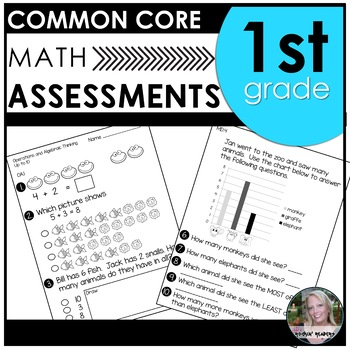# First Grade Common Core Math AssessmentsSubject
Resource Type
File Type
PDF (10 MB|78 pages)
Standards
\$6.00
• Product Description
• Standards

These common core assessments cover the standards for First Grade Math Common Core. Also included is a quick glance at all of the standards. The standard is marked on the assessments to let you know what standard is being covered. Some assessments deal with smaller numbers to allow teachers to use the assessments earlier in the school year before mastery is expected at the end of the year.

Included:

1) Operations and Algebraic Thinking

• The first assessment deals with numbers up to 10 (adding/subtracting etc.) and the second up to 20.

2) Number Operations in Base 10

• The first assessment deals with numbers up to 50 and the second numbers up to 120.

3) Measurement and Data-

• 2 assessments

4) Geometry

• 2 assessments

I also included a quick glance (4 pages) of all of the standards.

There is also 2 pages of individual assessments that cannot be assessed with paper and pencil. For example, asking students to tell you 10 more/10 less without having to count forward or backward.

Don't forget- leaving feedback earns you TPT credits toward free TPT purchases!

FOLLOW ME to be notified when new resources are uploaded AND I offer 50% off my products for the first 48 hours. It pays to follow.

Jennie

Partition circles and rectangles into two and four equal shares, describe the shares using the words halves, fourths, and quarters, and use the phrases half of, fourth of, and quarter of. Describe the whole as two of, or four of the shares. Understand for these examples that decomposing into more equal shares creates smaller shares.
Compose two-dimensional shapes (rectangles, squares, trapezoids, triangles, half-circles, and quarter-circles) or three-dimensional shapes (cubes, right rectangular prisms, right circular cones, and right circular cylinders) to create a composite shape, and compose new shapes from the composite shape.
Distinguish between defining attributes (e.g., triangles are closed and three-sided) versus non-defining attributes (e.g., color, orientation, overall size); build and draw shapes to possess defining attributes.
Determine the unknown whole number in an addition or subtraction equation relating three whole numbers. For example, determine the unknown number that makes the equation true in each of the equations 8 + ? = 11, 5 = ▯ - 3, 6 + 6 = ▯.
Understand the meaning of the equal sign, and determine if equations involving addition and subtraction are true or false. For example, which of the following equations are true and which are false? 6 = 6, 7 = 8 - 1, 5 + 2 = 2 + 5, 4 + 1 = 5 + 2.
Total Pages
78 pages